### The Masses of Stars

#### Chapter index in this window —   — Chapter index in separate window

This material (including images) is copyrighted!. See my copyright notice for fair use practices.

To determine the masses of stars, Kepler's third law is applied to the motions of binary stars---two stars orbiting a common point. The greater the combined mass of the two stars, the greater the gravity acceleration is, and, therefore, the smaller their orbital period. A majority of the several hundred billion stars in the Galaxy are in a system with two or more stars orbiting each other. Usually the binary stars are spectroscopic binary stars. A spectroscopic binary system is two stars orbiting a common point at too great a distance away from us to resolve the two stars individually, but whose binary nature is indicated in the periodic shift of their spectral lines as they orbit around each other. Spectroscopic binary stars are used because (a) there are a lot more far away stars than nearby ones and (b) more importantly, you can easily measure their speeds from the doppler shifted lines.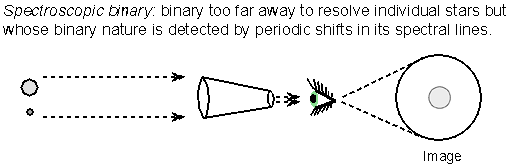Newton's form of Kepler's third law gives the combined mass of the two stars: (mass 1 + mass 2) = (separation distance)3/(orbital period)2 if you use solar mass units, the A.U. for the distance unit between the stars, and the time unit of years for the orbital period. The total distance between the two stars is used in Kepler's third law, but their individual distances from the common point they orbit is used to determine the stars' individual masses.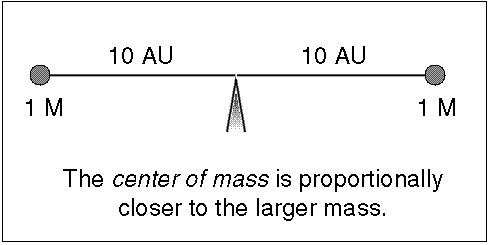Since stars have about the same mass (within a factor of 20), they both orbit around a common point, called the center of mass, that is significantly different from one of the star's center. The center of mass (C.M.) is the point where (mass star 1) × (C.M. distance 1) = (mass star 2) × (C.M. distance 2), or the point they would be balanced upon if the stars were on a stellar seesaw (it is the ``x'' in the figure below). The massive star is proportionally closer to the center of mass than the low-mass star and the massive star also moves proportionally slower than the low-mass star so its spectral lines have a smaller doppler shift.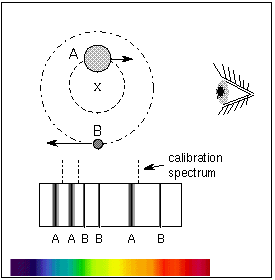Newton's Law of Gravity with Newton's second law of motion explains why this is. Both stars are experiencing the same gravity force between them [since (mass star 1) x (mass star 2) = (mass star 2) x (mass star 1)]. Given the same gravity force at work between the two stars, the lower-mass star will experience a greater acceleration than the more massive star (which star would be like Andre the Giant and which would be like Tom Thumb?). The lower-mass star moves faster and has a larger orbit. The more massive star's orbital speed is less and its orbit is smaller so that the two stars always stay on opposite sides of the center of mass. The two stars inside the binary system have the same orbital period around the center of mass.

Note of caution about comparisons: When comparing two separate binary systemS of the same separation distance, the two stars in the binary system that has larger combined mass will move faster than the two stars in the binary system with less combined mass. The larger combined mass binary has greater gravity force acting between the two stars. When comparing the two stars within a particular binary system, the larger mass star will move slower than the less massive star. The gravity force acting on the two stars within the binary is the same for both of the stars.

The distance travelled by an object = velocity × the time it takes. The distance travelled by the star is just the circumference of the orbit = 2p × the radius of a circular orbit and something similar for an elliptical orbit. Therefore, each star's C.M.-distance r = the star's velocity × the star's orbital period / (2p). This allows you to use the easily measured velocity in Kepler's third law and in the center of mass relations. The doppler shifts of the spectral lines are used to construct a radial velocity curve---a plot of the radial velocity (line of sight velocity) vs. time. The low-mass star will move proportionally faster than the massive star. Note that the two stars will have the same orbital period but the less massive star will have a larger orbit around the center of mass point, so it will have to move faster to compensate---both stars remain directly opposite each other as they orbit the center of mass with the center of mass always in between them.

Uncertainty arises, though, if the binary orbital plane is inclined to our line of sight by an angle amount i. In that very common case, the radial velocity = total velocity × Sin(inclination angle). The orbit's inclination angle i ranges from i=0° for a face-on orbit (viewing the orbit from directly above the system) to i=90° for an edge-on orbit (viewing the orbit along its plane). The inclination angle can be approximately determined from the plot of radial velocity vs. time. If the binary is an eclipsing binary, then you know that i=90° because you see them periodically pass in front of each other. Eclipsing binaries also allow us to accurately determine the diameters of stars (discussed in the next section). The radial velocity measurement technique has also been used to find planets around other stars and to locate black holes from the doppler shifts they produce in the visible stars they orbit around.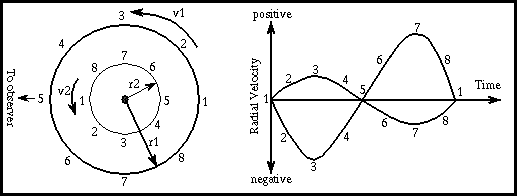Remember these rules:

1. Stars stay on the opposite side of the center of mass from each other.
2. The massive star moves slower than the low-mass star.
3. The center of mass is also the point where mass1 × velocity1 = mass2 × velocity2

Using the distance of the center of mass from each star, you can proportion out the total mass to each star. Here are the steps to figure out each star's mass:

1. Find the total mass (mass star A + mass star B) from Kepler's 3rd law.
2. Find the proportion of each star's mass to the total mass from the center of mass: (mass star A)/(mass star B) = (C.M. distance B)/(C.M. distance A) or (mass star A)/(mass star B) = (velocity star B)/(velocity star A). Note which star's values are on top of the fraction and which are on the bottom! Simplify the fraction down as far as possible.
3. If you set the mass of star A = (mass of star B)×(the fraction of the previous step) and substitute this for the mass of star A in the first step (Kepler's 3rd law step), you will find star B's mass = the total mass/(1 + the fraction from step 2).
4. Star A's mass = star B's mass × (the fraction from step 2).
5. Check that the proportions add up to the total mass!

(By the way, you can use this proportion idea in cooking if you need to have a 32-ounce mixture and the recipe calls for 3 parts sugar to 2 parts flour or if a recipe is for 6 people but you need to serve 8 people.) The masses of different types of stars are summarized in the Main Sequence Star Properties table below.

Use the UNL Astronomy Education program's Eclipsing Binary Simulator to further explore how the orbits of the stars in a binary star system change with different proportions of the masses (link will appear in a new window). "Eclipsing binaries" are discussed further in the next section of Astronomy Notes.

Even the smallest star's mass is much, much greater than a planet's so a "kilogram" is too tiny a unit of mass to use for the stars. Star masses are specified in units of solar mass---relative to the Sun (so the Sun has one solar mass of material). One solar mass is about 2 × 1030 kilograms.

### How do you do that?

Use the observed velocities in the figure below to find the individual masses of the stars. The stars have a measured period of 4/3 years and a separation distance of 4 A.U.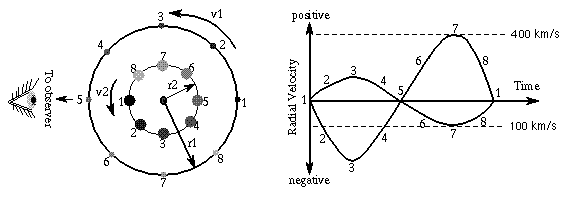Step 1:
Kepler's third law says the total mass = 43/(4/3)2 = 64/(16/9) = 36 solar masses.
Step 2:
I will let the massive star be ``star A''. So (mass star A)/(mass star B) = 400/100 = 4.
Step 3:
mass star B = 36 solar masses/(1 + 4) = 7.2 solar masses.
Step 4:
mass star A = mass star B × 4 = 28.8 solar masses.
Step 5: Check:
28.8 solar masses + 7.2 solar masses does equal 36 solar masses. This step makes sure you did not make an arithmetic error in the previous steps. If the sum does not equal the value in step 1, then re-check your math!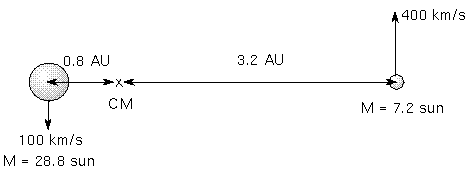One final caution: There is a difference between the mass of a star and its size (diameter)! Just because something is large in size (diameter) does not necessarily mean that it is more massive. Some stars are very large in diameter but have less mass than much smaller stars. For example, in the future, our Sun will become a red giant about 100 times larger in diameter than it is now, but it will have less mass than it does now. It will eventually become a white dwarf about the diameter of the Earth and that tiny white dwarf will be more massive than the much larger diameter ordinary "red dwarf M stars" discussed in a later section.

#### Vocabulary

center of mass radial velocity curve spectroscopic binary
solar mass

#### Formulae

• Center of mass distance: mass star A / mass star B = distance B / distance A, where the distances are each measured from the center of mass. Notice which star's distance is in the top of the fraction!
• separation distance = distance A + distance B, where the distances are each measured from the center of mass.
• Center of mass velocity: mass star A / mass star B = velocity star B / velocity star A. Notice which star's velocity is in the top of the fraction!
• Kepler's 3rd law: (mass star A + mass star B) = (separation distance)3 / (orbital period)2 if use solar mass units, A.U. for the distance unit between the stars, and the time unit of years for the orbital period.

#### Review Questions

1. How the masses of stars found? What kind of star systems are used and which famous law of orbital motion is used?
2. How many times closer to the center of mass is the massive star than the low-mass star?
3. How do you use the radial velocity curve to find the mass proportions and separation distance? How much faster or slower does the low-mass star move than the massive star?
4. Three binary systems with a separation of 2 A.U. between the two stars in each system. System (1) has the two stars orbiting the center of mass in 1 year, system (2) has the two stars orbiting the center of mass in 5 months, and system (3) has the two stars orbiting the center of mass in 2 years. Put the binary systems in the correct order by increasing total mass (least massive first and ignore the inclination angle i).
5. Star A is 0.2 A.U. from the center of mass and its companion star B is 0.6 A.U. from the center of mass. Which star is more massive?
6. If the two stars in the previous question have orbital periods of 0.35777 years, what are the individual masses of the two stars? (Hint: find their combined mass from Kepler's third law and then use their relative center of mass distances to find how many times more massive one star is than the other.)
7. Use the radial velocity curve graph in the text above. Assume that star A reaches a velocity of 90 kilometers/second and star B reaches only 10 kilometers/second. If the separation distance = 10 A.U., and the orbital period = 10 years, what is the combined mass of the two stars? From the center of mass relation also find (star A mass)/(star B mass) and their individual masses.
8. Which star system(s) would you be able to measure the radial velocity: (a) stars orbiting in a plane that is along our line of sight (i=0°); (b) stars orbiting in a plane that is perpendicular to our line of sight (face-on, i=90°); (c) stars orbiting in a plane with i=30°.

#### Go to Astronomy Notes home

last updated: June 12, 2010